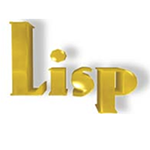# LISP数据类型

LISP数据类型可分类为：

• 标量类型 - 例如，数字类型，字符，符号等。

• 数据结构 - 例如，列表，向量，比特向量和字符串。

typep谓词用于发现一个对象是否属于一个特定的类型。

type-of函数，返回给定对象的数据类型的类型。

## 在LISP类型说明符

 array fixnum package simple-string atom float pathname simple-vector bignum function random-state single-float bit hash-table ratio standard-char bit-vector integer rational stream character keyword readtable string [common] list sequence [string-char] compiled-function long-float short-float symbol complex nill signed-byte t cons null simple-array unsigned-byte double-float number simple-bit-vector vector

```(setq x 10)
(setq y 34.567)
(setq ch nil)
(setq n 123.78)
(setq bg 11.0e+4)
(setq r 124/2)
(print x)
(print y)
(print n)
(print ch)
(print bg)
(print r)```

```10
34.567
123.78
NIL
110000.0
62```

```(setq x 10)
(setq y 34.567)
(setq ch nil)
(setq n 123.78)
(setq bg 11.0e+4)
(setq r 124/2)
(print (type-of x))
(print (type-of y))
(print (type-of n))
(print (type-of ch))
(print (type-of bg))
(print (type-of r))```

```(INTEGER 0 281474976710655)
SINGLE-FLOAT
SINGLE-FLOAT
NULL
SINGLE-FLOAT
(INTEGER 0 281474976710655)```